Function Repository Resource:

# GeneralizedFiedlerMatrix

Generate the generalized Fiedler companion matrix of a univariate polynomial

Contributed by: Jan Mangaldan
 ResourceFunction["GeneralizedFiedlerMatrix"][poly,x] gives the Fiedler companion matrix of poly, treated as a polynomial in x. ResourceFunction["GeneralizedFiedlerMatrix"][perm,poly,x] gives the generalized Fiedler companion matrix associated with the permutation perm.

## Details and Options

The Fiedler companion matrix of a univariate polynomial is a pentadiagonal matrix whose characteristic polynomial is a scalar multiple of the original polynomial.
The generalized Fiedler companion matrix of a univariate polynomial is a sparse matrix whose characteristic polynomial is a scalar multiple of the original polynomial.
The permutation perm can be in a disjoint cycle or permutation list representation.

## Examples

### Basic Examples (2)

Generate a Fiedler companion matrix:

 In:=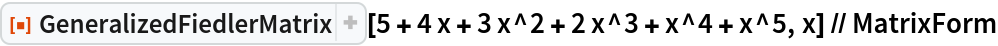Out=The characteristic polynomial of this matrix is a constant multiple of the original polynomial:

 In:=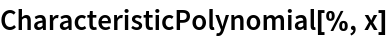Out=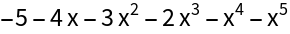### Scope (3)

Generate the regular Fiedler matrix:

 In:=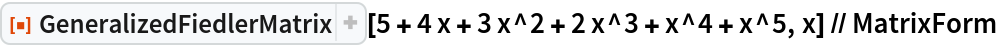Out=Use a different permutation:

 In:=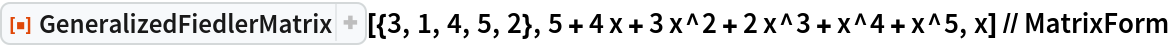Out=Use a disjoint cycle representation of a permutation:

 In:=Out=### Applications (2)

Numerically find the roots of a polynomial by computing the eigenvalues of its Fiedler companion matrix:

 In:=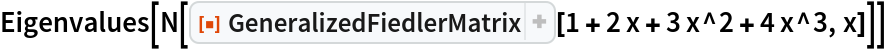Out=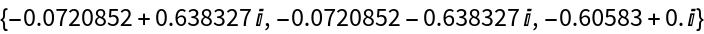Compare with the result of NSolve:

 In:=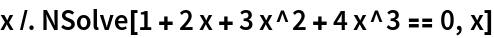Out=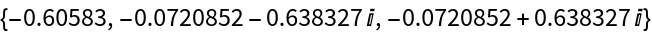### Properties and Relations (2)

Different permutations may yield the same companion matrix:

 In:=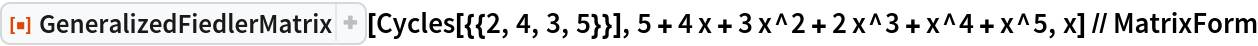Out=In:=Out=The Frobenius companion matrix (with polynomial coefficients placed on the first row) is a special case of the generalized Fiedler matrix:

 In:=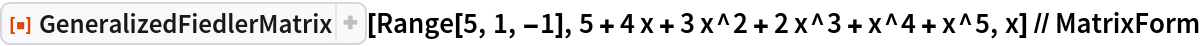Out=## Version History

• 1.0.0 – 31 December 2020